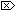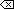The Open Group Base Specifications Issue 6
IEEE Std 1003.1-2001

#### NAME

llround, llroundf, llroundl, - round to nearest integer value

#### SYNOPSIS

```#include <math.h> long long llround(double x); long long llroundf(float x); long long llroundl(long double x); ```

#### DESCRIPTION

[CX]The functionality described on this reference page is aligned with the ISO C standard. Any conflict between the requirements described here and the ISO C standard is unintentional. This volume of IEEE Std 1003.1-2001 defers to the ISO C standard.These functions shall round their argument to the nearest integer value, rounding halfway cases away from zero, regardless of the current rounding direction.

An application wishing to check for error situations should set errno to zero and call feclearexcept(FE_ALL_EXCEPT) before calling these functions. On return, if errno is non-zero or fetestexcept(FE_INVALID | FE_DIVBYZERO | FE_OVERFLOW | FE_UNDERFLOW) is non-zero, an error has occurred.

#### RETURN VALUE

Upon successful completion, these functions shall return the rounded integer value.

[MX]If x is NaN, a domain error shall occur, and an unspecified value is returned.

If x is +Inf, a domain error shall occur and an unspecified value is returned.

If x is -Inf, a domain error shall occur and an unspecified value is returned.

If the correct value is positive and too large to represent as a long long, a domain error shall occur and an unspecified value is returned.

If the correct value is negative and too large to represent as a long long, a domain error shall occur and an unspecified value is returned.#### ERRORS

These functions shall fail if:

Domain Error
[MX]The x argument is NaN or ±Inf, or the correct value is not representable as an integer.

If the integer expression (math_errhandling & MATH_ERRNO) is non-zero, then errno shall be set to [EDOM]. If the integer expression (math_errhandling & MATH_ERREXCEPT) is non-zero, then the invalid floating-point exception shall be raised.The following sections are informative.

None.

#### APPLICATION USAGE

On error, the expressions (math_errhandling & MATH_ERRNO) and (math_errhandling & MATH_ERREXCEPT) are independent of each other, but at least one of them must be non-zero.

#### RATIONALE

These functions differ from the llrint() functions in that the default rounding direction for the llround() functions round halfway cases away from zero and need not raise the inexact floating-point exception for non-integer arguments that round to within the range of the return type.

None.